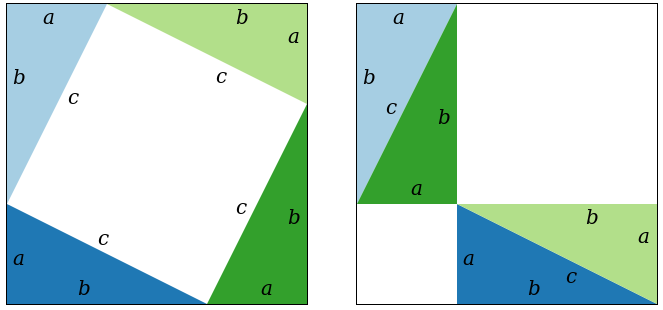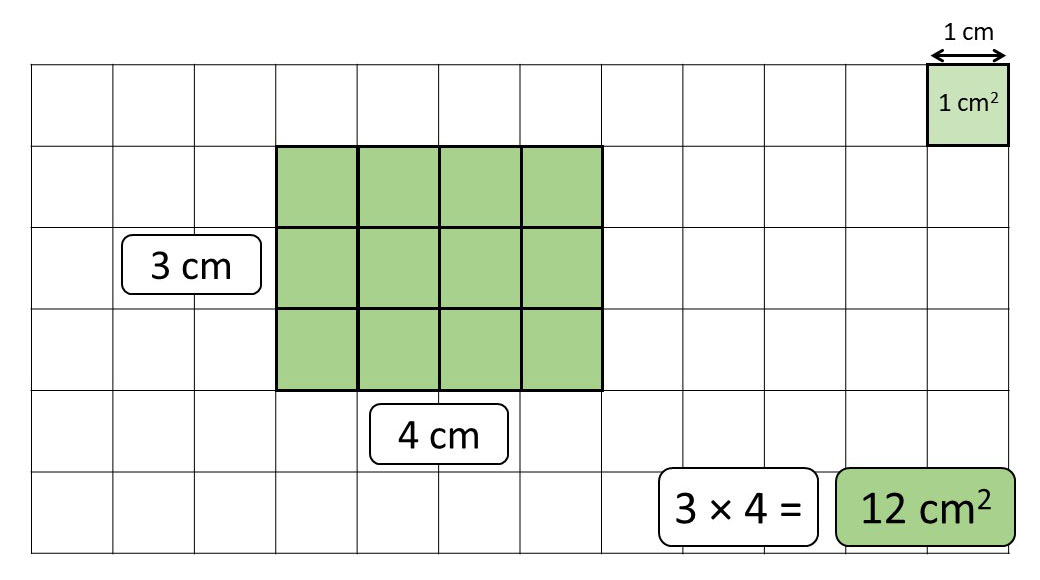# 【已解决】对发现无理数过程的逻辑严谨性的疑惑35

In order to calculate the area of a plane figure we choose as the unit of area a square whose sides are of unit length. If the unit of length is the inch, the corresponding unit of area will be the square inch; i.e. the square whose sides are of length one inch. On the basis of this definition it is very easy to calculate the area of a rectangle. If $p$ and $q$ are the lengths of two adjacent sides measured in terms of the unit of length, then the area of the rectangle is $p q$ square units, or, briefly, the area is equal to the product $p q .$ This is true for arbitrary $p$ and $q,$ rational or not. For rational $p$ and $q$ we obtain this result by writing $p=m / n$ $q=m^{\prime} / n^{\prime},$ with integers $m, n, m^{\prime}, n^{\prime} .$ Then we find the common measure $1 / N=1 / n n^{\prime}$ of the two edges, so that $p=m n^{\prime} \cdot 1 / N, q=$ $n m^{\prime} \cdot 1 / N .$ Finally, we subdivide the rectangle into small squares of side $1 / N$ and area $1 / N^{2} .$ The number of such squares is $n m^{\prime} \cdot m n^{\prime}$ and the total area is $n m^{\prime} m n^{\prime} \cdot 1 / N^{2}=n m^{\prime} m n^{\prime} / n^{2} n^{\prime 2}=m / n \cdot m^{\prime} / n^{\prime}=p q$

If $p$ and $q$ are irrational, the same result is obtained by first replacing $p$ and $q$ by approximate rational numbers $p_{r}$ and $q_{r}$ respectively, and then letting $p_{r}$ and $q_{r}$ tend to $p$ and $q$. 下面是中文叙述：7

1. The Irrationals, Julian Havil, p19↩︎

2. Introduction to Calculus and Analysis Volume I, Reprint of the 1989 edition, Richard Courant, Fritz John, p5↩︎

3. https://en.wikipedia.org/wiki/Pythagorean_theorem↩︎

4. What Is Mathematics? Second Edition, Courant and Robbins, p399↩︎

5. https://www.goteachmaths.co.uk/area-of-a-rectangle/↩︎

6. Richard Courant, Fritz John, Introduction to Calculus and Analysis Volume I, Reprint of the 1989 edition, p120↩︎

7. https://www.goteachmaths.co.uk/area-of-a-rectangle/↩︎

8. What Is Mathematics? Second Edition, Courant and Robbins, p399-400↩︎